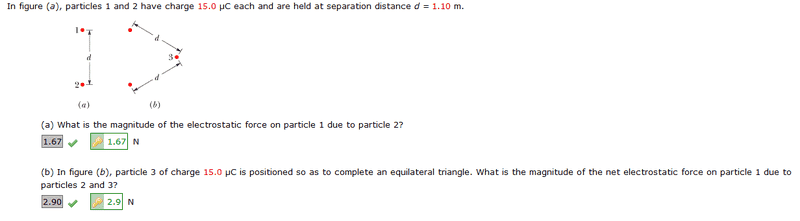# Understanding Trig In Force Diagrams

PhysicsReaper
Hello,

I have the following problem part (b) which I already solved as you can see in the attached image. So I am not asking homework questions, I merely reviewing my homework for a better understanding for the test. I obtained the answer from a friend showing me his method. However, I am studying and a listed solution was the following:

F_y = 2*(k*(q^2/r^2))cos(30)

I've drawn out a force diagram but have no idea how, they have obtained the cosign. In the listed solution they state use oriented the y-axis such that it bisects charges q2 and q3.Homework Helper
Gold Member
There are two forces acting on point 1. By symetry the result of these two forces will act along a line drawn through point 1 and a point mid way between 2 and 3. See diagram.

So work out the component of the two forces pointing in that direction.

Consider the triangle on the right.

Cos(30) = Fr/F

so

Fr = F Cos(30)

That's not the whole solution obviously, just where the cos(30) comes from. The angle doesn't come from the direction of the result per se, it comes from the direction of the result in relation to the forces. eg If you rotate the triangle/problem drawing 30 degrees so the resulting force is vertical (on the y-axis) the answer will still contain cos(30).

#### Attachments

Last edited:
PhysicsReaper
Thank you very much! And because of symmetry is why it's multiplied by 2?